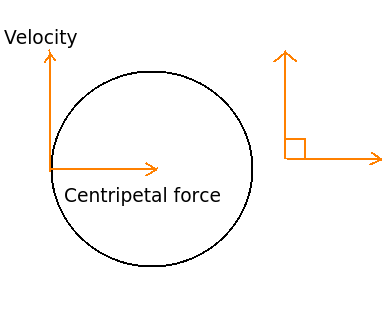# Numerical - Work done by centripetal force - Mechanics

Q.  If a particle of mass 5 kg moves uniformly along a circle of radius 10 m at 10 m/s, then what is the work done by centripetal force during its one revolution?
- Published on 21 Sep 15

a. 0.5 kNm
b. zero
c. infinity
d. none of the above

#### Discussion

• Sravanthi   -Posted on 15 Dec 15
- Centripetal force causes an object to move with uniform speed along a circular path and counteracts the centrifugal force.

- Centrifugal force causes an object to move away from the centre of curvature.

- Work done in centripetal is zero because no displacement is observed in this direction. When force and displacement are collinear only then work is done. But force and displacement are not collinear when centripetal force acts on an object.- Work done = Force x displacement x cos θ

- The angle between force and displacement is 90° as shown in the diagram.

Work done = Force x displacement x cos 90

Since, cos 90 = 0

- Work done = Force x displacement x 0

Therefore, work done is equal to zero.

## ➨ Post your comment / Share knowledgeEnter the code shown above:

(Note: If you cannot read the numbers in the above image, reload the page to generate a new one.)